# Precalculus : Double-Angle Identities

## Example Questions

### Example Question #1 : Double Angle Identities

Find the exact value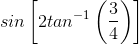.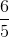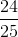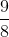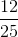Explanation:

By the double angle formula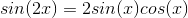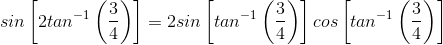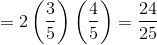### Example Question #1 : Double Angle Identities

Find the exact value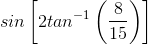.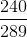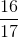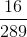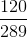Explanation:

By the double angle formula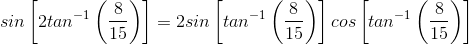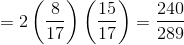### Example Question #1 : Double Angle Identities

Find the exact value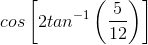.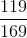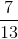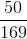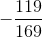Explanation:

By the double-angula formula for cosine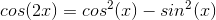For this problem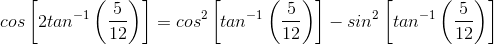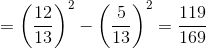### Example Question #1 : Double And Half Angle Identities

Find the exact value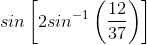.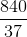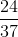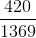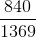Explanation:

By the double-angle formula for the sine functionwe have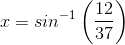thus the double angle formula becomes,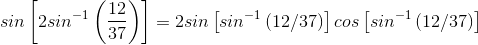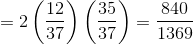### Example Question #3 : Double Angle Identities

If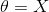, which of the following best represents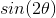?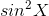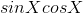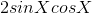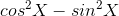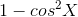Explanation:

The expressionis a double angle identity that can also be rewritten as: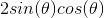Replace the value of theta for.

The correct answer is: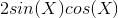### Example Question #4 : Double Angle Identities

Which expression is equivalent to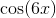?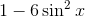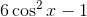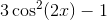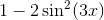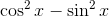Explanation:

The relevant trigonometric identity is: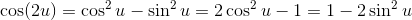In this case, "u" issince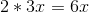.

The only one that actually follows this is### All Precalculus Resources